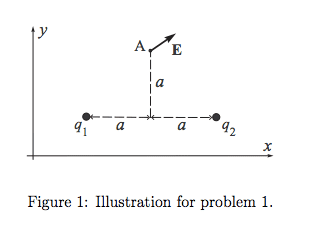# Find the unknown charges q1 and q2

## Homework Statement

The geometrical positions of point-like charges and point A situated in the xy-plane in terms of the length parameter a. The vector of electric field E at point A is shown schematically and measured as E = Exi + Eyj (that is, both Ex and Ey are given). If possible, find the unknown charges q1 and q2.

**E_2 is the electric field with respect to q2. Not shown in figure. **
**E_1 is the electric field with respect to q1. (Same direction as E) **

**k_e is Coulomb's constant.**## Homework Equations

E[/B] = Exi + Eyj
Ex = E_1*cos(45°) - E_2*cos(45°)
Ey = E_1*sin(45°) + E_2*sin(45°)
E = F/q = (k_e*q)/r^2

## The Attempt at a Solution

E_1 [/B]= (k_e*q1)/(2a)^2 = (k_e*q1)/(4a^2)

E_2 = (k_e*q2)/(2a)^2 = (k_e*q2)/(4a^2)

Ex = (E_1 - E_2)*2/sqrt(2)

Ey = (E_1 + E_2)*2/sqrt(2)

E
= 2/sqrt(2)*(E_1 - E_2) i + 2/sqrt(2)*(E_1 + E_2) j = 2/sqrt(2)*(k_e/(4a^2))*(q1 - q2) i + 2/sqrt(2)*(k_e/(4a^2))*(q1 + q2)

I am stuck here; I'm not sure if I've been going about this correctly or what steps to take next.

Thank you.

SammyS
Staff Emeritus
Homework Helper
Gold Member

## Homework Statement

The geometrical positions of point-like charges and point A situated in the xy-plane in terms of the length parameter a. The vector of electric field E at point A is shown schematically and measured as E = Exi + Eyj (that is, both Ex and Ey are given). If possible, find the unknown charges q1 and q2.

**E_2 is the electric field with respect to q2. Not shown in figure. **
**E_1 is the electric field with respect to q1. (Same direction as E) **

**k_e is Coulomb's constant.**

View attachment 102522

## Homework Equations

E[/B] = Exi + Eyj
Ex = E_1*cos(45°) - E_2*cos(45°)
Ey = E_1*sin(45°) + E_2*sin(45°)
E = F/q = (k_e*q)/r^2

## The Attempt at a Solution

E_1 [/B]= (k_e*q1)/(2a)^2 = (k_e*q1)/(4a^2)

E_2 = (k_e*q2)/(2a)^2 = (k_e*q2)/(4a^2)

Ex = (E_1 - E_2)*2/sqrt(2)

Ey = (E_1 + E_2)*2/sqrt(2)

E
= 2/sqrt(2)*(E_1 - E_2) i + 2/sqrt(2)*(E_1 + E_2) j = 2/sqrt(2)*(k_e/(4a^2))*(q1 - q2) i + 2/sqrt(2)*(k_e/(4a^2))*(q1 + q2)

I am stuck here; I'm not sure if I've been going about this correctly or what steps to take next.

Thank you.
Hello JessieS , Welcome to PF !

Are you given any numerical values, particularly any for Ex and Ey ?

Hello JessieS , Welcome to PF !

Are you given any numerical values, particularly any for Ex and Ey ?

Thank you!

And no I am not. I am supposed to just use Ex and Ey as variables.

SammyS
Staff Emeritus
Homework Helper
Gold Member
Thank you!

And no I am not. I am supposed to just use Ex and Ey as variables.
OK.

You are on the right track.

First, at least one error. What is the distance from q1 to A and q2 to A. The square of each of those distances is 2a2, not 4a2 .

I suggest that you keep Ex and Ey separate, rather than lumping them together into one big vector expression.

You have that Ex = C⋅(q1 - q2) and Ey = C⋅(q1 + q2) , where the coefficient, C is made up of all that stuff in your equation.

OK.

You are on the right track.

First, at least one error. What is the distance from q1 to A and q2 to A. The square of each of those distances is 2a2, not 4a2 .

I suggest that you keep Ex and Ey separate, rather than lumping them together into one big vector expression.

You have that Ex = C⋅(q1 - q2) and Ey = C⋅(q1 + q2) , where the coefficient, C is made up of all that stuff in your equation.

Ok, so could I do this?

Since
Ex = 2/√2*(q1 - q2)*(ke/(2a2))

Ey = 2/√2*(q1 + q2)*(ke/(2a2))

Then
(q1 - q2) = (Ex * a2*√2)/ke
+ (q1 + q2) = (Ey * a2*√2)/ke
--------------------------------
q1 = (a2*√2)/ke * (Ex + Ey)

So then

q2 = (a2*√2)/ke * (Ey - Ex)

Is that correct?

•SammyS
SammyS
Staff Emeritus
Homework Helper
Gold Member
Ok, so could I do this?

Since
Ex = 2/√2*(q1 - q2)*(ke/(2a2))

Ey = 2/√2*(q1 + q2)*(ke/(2a2))

Then
(q1 - q2) = (Ex * a2*√2)/ke
+ (q1 + q2) = (Ey * a2*√2)/ke
--------------------------------
q1 = (a2*√2)/ke * (Ex + Ey)

So then

q2 = (a2*√2)/ke * (Ey - Ex)

Is that correct?
Yes. That's it.

Subtracting should give q2.

Yes. That's it.

Subtracting should give q2.

Thank you for your help!